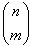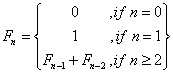## 2008年8月21日 星期四

### BigDump - 載入大檔案的sql檔到MySQL

2. MySQL Tools - MySQL Query Browser
Open SQL檔時，整個畫面都沒出現東西，預估是檔案過大。所以不可行

3. 用命令提示字元，直接連結資料庫匯入
mysql -u root -p myDB < myDB.sql

4. BigDump: Staggered MySQL Dump Importer v0.29b
http://www.ozerov.de/bigdump.php

## 2008年8月11日 星期一

### Binomial Coefficient (二項式係數) + Pascal (巴斯卡三角形)(1) Write a recursive C program

(2)= ?
(3) 共呼叫此函數幾次 (連同第一次呼叫)?

Ans:
(1)
`int Bin(int n, int m) {  if (n==m||m==0) {     return 1;  } else {     return Bin(n-1, m)+Bin(n-1, m-1);  }}`

(2)
Bin(5,3) = Bin(4,3)+Bin(4,2)
= Bin(3,3)+Bin(3,2)+Bin(3,2)+Bin(3,1)
= 1+Bin(2,2)+Bin(2,1)+Bin(2,2)+Bin(2,1)+Bin(2,1)+Bin(2,0)
= 1+1+Bin(1,1)+Bin(1,0)+1+Bin(1,1)+Bin(1,0)
+Bin(1,1)+Bin(1,0)+1
= 1+1+1+1+1+1+1+1+1+1
= 10

(3)

=============

Pascal 巴斯卡三角形
n 為 row, r 為 column
nC0 = 1
nCr = [(n-r+1)/r] * nCr-1

`int main(){int N=4;int n,r,i;    for (n=0;n<=N;n++) {        for (r=0;r<=n;r++) {                    long nCr = 1;            for(i=1;i<=r;i++) {                nCr = nCr * (n-i+1)/i;            }            cout << nCr;        }        cout << "\n";    }    system ("pause");    return 0 ; }`

1
11
121
1331
14641

## 2008年8月8日 星期五

### Fibonacci Number (費氏數列)(1) Write a recursive finction in C
(2) Fib (5) = ?
(3) Fib(5)求值過程中, 共呼叫幾次Fib Function?

Ans:

(1)
`int Fib(int n) { if(n==0) return 0;    else if(n==1) return 1;       else return Fib(n-1)+Fib(n-2);}`

(2)
Fib(5) = Fib(4) + Fib(3)
= Fib(3) + Fib(2) + Fib(2) + Fib(1)
= Fib(2) + Fib(1) + Fib(1) + Fib(0) + Fib(1) + Fib(0) + 1
= Fib(1) + Fib(0) + 1 + 0 + 1 + 0 + 1
= 1+0+1+0+1+0+1
= 5

(3)

## 2008年8月6日 星期三

### Ruby on Rails : Step by Step建立線上書籤

Step 1. 安裝Rails
gem install rails--include-dependencies

Step 2. 建立專案(預設是SQLLite，所以要自行指定資料庫，也可以直接打rails看詳細指令用法)
rails bookmarker -d mysql

Step 6. 使用Migration 建立資料表
rake migrate 會有錯誤，改用 rake db:migrate

Step 7. 使用Scaffold方法 產生頁面
bookmark_controller.rb在.\app\controllers

Step 8. 啟動內建伺服器
ruby script\server

undefined method `scaffold' for BookmarkController:Class
undefined method `paginate' for #

ruby script/plugin install scaffolding

ruby script/plugin install http://tools.assembla.com/svn/breakout/breakout/vendor/plugins/classic_pagination/

Step 10. 透過Scaffold 產生程式碼 (原文多一個bookmark)
Ruby script/generate scaffold bookmark

CRUD - Create, Read, Update, Delete